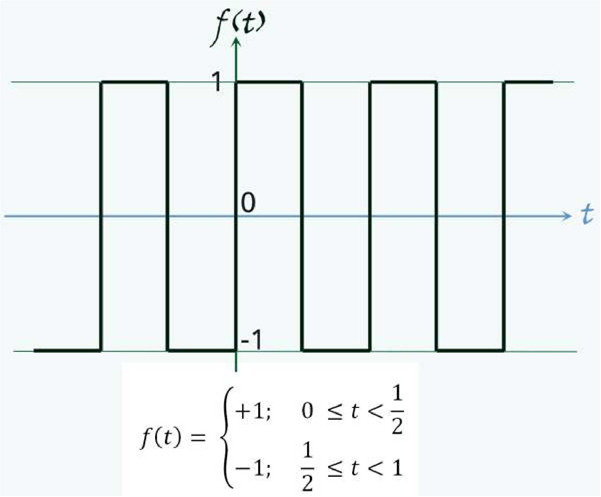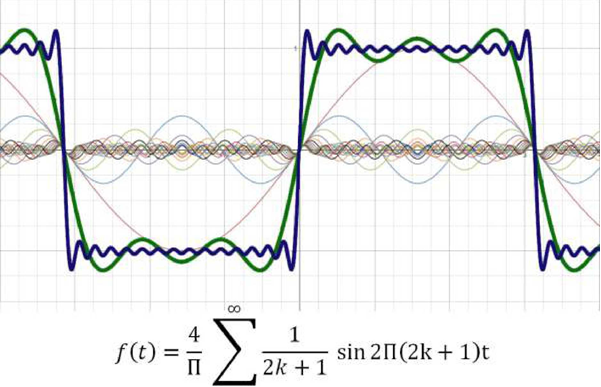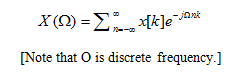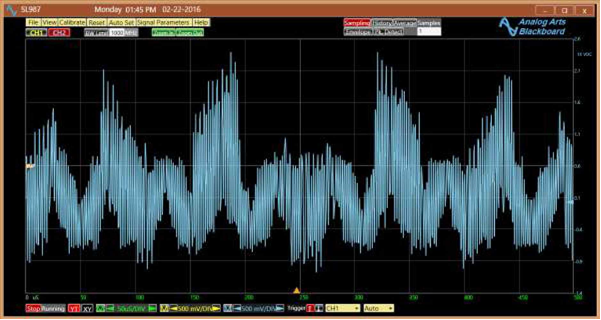News/Events

# FFT: EQUATIONS AND HISTORY

## The FFT (Fast Fourier Transform) is rightfully regarded as the most important numerical algorithm of our lifetime. Whether it's used to monitor signals coming from the depths of the earth or search for heavenly life forms, the algorithm is widely used in all scientific and engineering fields. But,what exactly is an FFT? Why does it matter in signal-processing projects?

Named after the late 18th century French mathematician Jean-Baptiste Joseph Fourier, the Fourier Transform is a mathematical operation that converts a signal from the time (spatial) domain to the frequency domain. According to Fourier theorem, a signal is a composition of a number of sinusoidal functions with given amplitude, frequency, and phase. The Fourier series equation formulates the theorem for a periodic signal,x(t):For a sine wave, there is only one coefficient. If, however, the signal contains an infinite number of frequencies, such as is the case for an ideal square wave, a finite N results in an error. Figures 1 and 2 compare the original signal with its representation based on Fourier series coefficients for N=3 (green signal) and N=15 (blue signal.) As we can see, the accuracy of the transformation depends on the nature of the signal and the number of terms in the series.Figure 1. An ideal square wave function.Figure 2. The Fourier series reconstruction of the square wave for  and . Plot calculated by: https://www.desmos.com/calculator.

Most signals, however, aren't periodic. Nonperiodic signals don't have a Fourier series. This fact greatly disappointed Fourier, who needed almost 20 years to develop a general formula that would work for any function. Now, any signal could be transformed from the time domain to the frequency domain by the following equation:This equation is much more than just a mere mathematical identity. It portrays the building blocks of signals, each block individually. That is, no matter how small or big the components of a signal are, they will show up in the ingredient list provided by the transformation. Consider a complex signal composed of a sine wave and a sinc function as shown in Figure 3.Figure 3

The time-domain representation of the signal provides nothing useful. Its amplitude frequency spectrum (Figure 4) clearly shows its composition. Note that the components of the interest can individually be analyzed. That is the power of the transform.Figure 4

The formula was, indeed, great. But, it would take forever to perform the transform. The number of operations required to process this equation as written is proportional to  Clearly, the issue becomes much worse for higher values of  as the number of operations quickly grows to impractical levels. Early computers took hundreds of hours to make a simple transform with respect to today's standards. Due to this, ever since 1805, there have been attempts to improve the efficiency of the algorithm. That year, an effective method of Fourier transformation was invented by Carl Friedrich Gauss. But, it remained obscure for another 160 years.

In 1965, James Cooley of IBM and John Tukey of Princeton rediscovered the algorithm an popularized it. Their work dramatically reduced the number of operations. The reduction is especially notable when N is equal to a power of 2. For this case, the number of operations is proportional to  The algorithm became known as FFT shortly after its introduction. It immediately revolutionized the Fourier transformation of signals. For a 64 kpoint FFT, the algorithm is remarkably 4000 times faster than the original method. The algorithm has seen several modifications since its release. In January 2000, it was included in Top 10 Algorithms of 20th century.

With the advent of the digital signal processing, the FFT found a new importance. Several companies such as Texas Instruments and Analog Devices introduced dedicated FFT processors. Once the signal exits the analog domain through an analog to digital converter, the possibilities are endless.Figure 5, Signals from Arecibo Observatory in Puerto Rico are analyzed in search of alien intelligence..

Numerous applications benefit from the FFT, with the communication field particularly enjoying its power. Radar, cellular phones, speech processing, and SETI (search for extraterrestrial intelligence) are a few applications, which extensively use FFT.

Applications for FFT encompass all walks of life. Whether it is a patient monitoring device, a synthesizer, or a signal analyzer, be sure Fourier is hard at work. These days, most signals go nowhere without Fourier's blessing.

Source: RSI

## Others

@ 2015 Tecotec Group